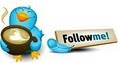#How to handle count of data with “valid to/from” dates in DAX• ### Question

•I have a problem making a proper DAX formular for handling “facts” with valid to/from dates in Excel 2013 PowerPivot.

My goal is to calculate (distinct) counts per period (date, week, month) of my ”fact data”, but the challenge is that the “facts” have valid from/to dates, and are not stored per individual date (like all the normal PowerPivot examples I can find).

I have a “dates” table that looks something like this:My (exemplified) “fact” table looks like this:I have not created any relations between the two tables, as I am not interested in doing queries on specific to/from dates in the fact table, but instead I want to be able to answer questions like:

• Total number of team memberships for a given date in january
• How many member of the blue team was there in the 2<sup>nd</sup> of January?
• Show me the number of teams that member A was on per week

I have worked on several DAX formulars in Excel to calculate this, but I just cannot seem to get it to work.

The last one I have made is this one, added as a calculated column on the dates table, but it returns 9 for all records (my goal was to get it to return the number of fact records covering the given date):

=Calculate(

DISTINCTCOUNT( Data[Id]);

FILTER(

Data;

COUNTROWS(

FILTER(

VALUES(Calendar[Date]);

Data[DateFrom] <= Calendar[Date] && Data[DateTo] >= Calendar[Date]

)

) > 0

)

)

Can anyone point me in the right direction here: How do you handle lookups based on dates to data with valid to/from periods and not specific dates?

Kind regards,

Rolf

Friday, November 16, 2012 9:55 AM

•EDIT : I used the same formula that you wrote and it worked for me. However, I had to use the DateInt columns instead of the actual date both in the fact as well as the Date dimension. You can notice that in the image also below that I am using DateInt columns and not actual date columns.

I used the approach that Chris Webb suggested -

http://cwebbbi.wordpress.com/2010/01/12/solving-the-%E2%80%98events-in-progress%E2%80%99-problem-in-dax-part-two-aggregating-dates-and-multiselect/

And here is the resultAnd here is the formula I used

```=Calculate(CountRows(Fct),
FILTER(Fct,
COUNTROWS(FILTER(VALUES('Date'[DateInt]),  ('Date'[DateInt]>=VALUE(LEFT(Fct[DtFrmTo], 8))) && ('Date'[DateInt]<=VALUE(RIGHT(Fct[DtFrmTo],8)))))
>0))```
Note that [DtFrmTo] is my concatenated columns of the DateInts of DateFrom and DateTo

Cheers,
Jason | www.SqlJason.comFriday, November 16, 2012 4:49 PM
•I am not sure that Jason's formula is correct - trying it on single days it returns strange numbers.

Moreover, there is a more performant solution that can be obtained by using this approach - please note that the FILTER functions operate on a small number of distinc values and not directly on the fact table, which is the reason why performance are better.

=

CALCULATE (

COUNTROWS ( Fact ),

FILTER ( VALUES ( Fact[DateFrom] ), Fact[DateFrom] <= MIN ( Calendar[Date] ) ),

FILTER ( VALUES ( Fact[DateTo] ), Fact[DateTo] >= MAX ( Calendar[Date] ) )

)

Marco Russo http://ssasworkshop.com http://www.sqlbi.com http://sqlblog.com/blogs/marco_russo

• Marked as answer by Tuesday, November 20, 2012 8:11 PM
Friday, November 16, 2012 8:27 PM
•As far as I can tell, your expression delivers the intended result, when used as a measure. That is, if you intened on calculating the number of facts in your Data table for the "current" time intervall.

The Data Specialist (Blog)

Friday, November 16, 2012 2:52 PM

### All replies

•As far as I can tell, your expression delivers the intended result, when used as a measure. That is, if you intened on calculating the number of facts in your Data table for the "current" time intervall.

The Data Specialist (Blog)

Friday, November 16, 2012 2:52 PM
•EDIT : I used the same formula that you wrote and it worked for me. However, I had to use the DateInt columns instead of the actual date both in the fact as well as the Date dimension. You can notice that in the image also below that I am using DateInt columns and not actual date columns.

I used the approach that Chris Webb suggested -

http://cwebbbi.wordpress.com/2010/01/12/solving-the-%E2%80%98events-in-progress%E2%80%99-problem-in-dax-part-two-aggregating-dates-and-multiselect/

And here is the resultAnd here is the formula I used

```=Calculate(CountRows(Fct),
FILTER(Fct,
COUNTROWS(FILTER(VALUES('Date'[DateInt]),  ('Date'[DateInt]>=VALUE(LEFT(Fct[DtFrmTo], 8))) && ('Date'[DateInt]<=VALUE(RIGHT(Fct[DtFrmTo],8)))))
>0))```
Note that [DtFrmTo] is my concatenated columns of the DateInts of DateFrom and DateTo

Cheers,
Jason | www.SqlJason.comFriday, November 16, 2012 4:49 PM
•I am not sure that Jason's formula is correct - trying it on single days it returns strange numbers.

Moreover, there is a more performant solution that can be obtained by using this approach - please note that the FILTER functions operate on a small number of distinc values and not directly on the fact table, which is the reason why performance are better.

=

CALCULATE (

COUNTROWS ( Fact ),

FILTER ( VALUES ( Fact[DateFrom] ), Fact[DateFrom] <= MIN ( Calendar[Date] ) ),

FILTER ( VALUES ( Fact[DateTo] ), Fact[DateTo] >= MAX ( Calendar[Date] ) )

)

Marco Russo http://ssasworkshop.com http://www.sqlbi.com http://sqlblog.com/blogs/marco_russo

• Marked as answer by Tuesday, November 20, 2012 8:11 PM
Friday, November 16, 2012 8:27 PM
•Hi guys,

Thank you all for the quick questions, it looks very promising. Unfortunately I am out of the office now, but I will test these things out as soon as I have access to the system again. Have a nice weekend and thanks for the help so far.

// Rolf

Friday, November 16, 2012 8:34 PM
•Thank you for the replies. I have now had a chance to test the formulars and they work fine. I actually think that the biggest problem was that I added the formular as a calculated column and not a measurement. Changing this gave much better results to all my tests.

Tuesday, November 20, 2012 8:15 PM